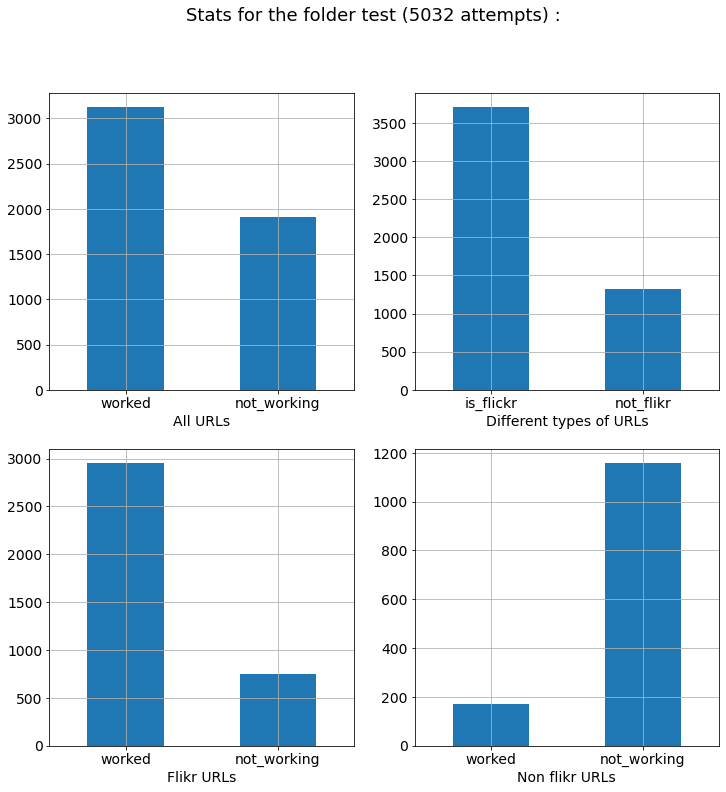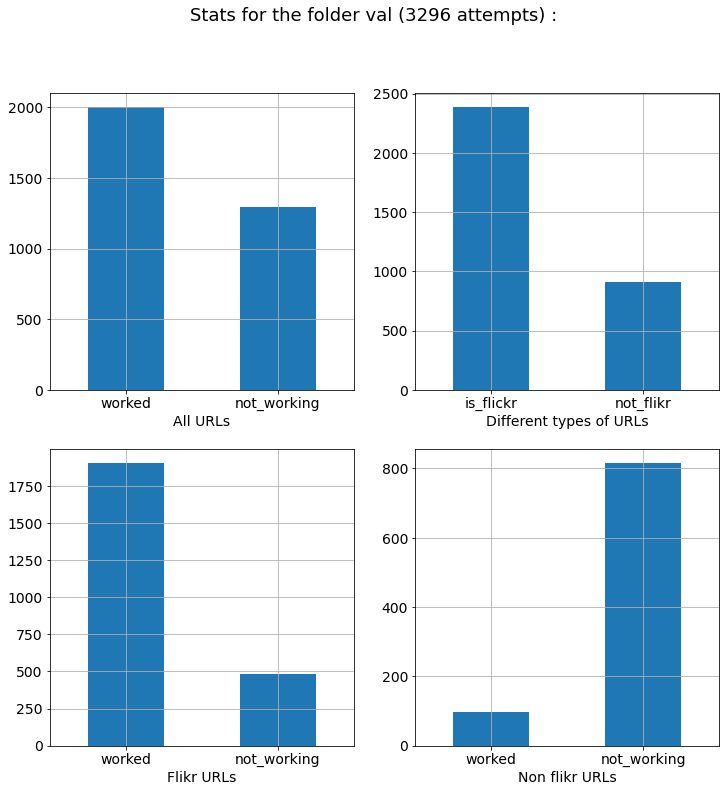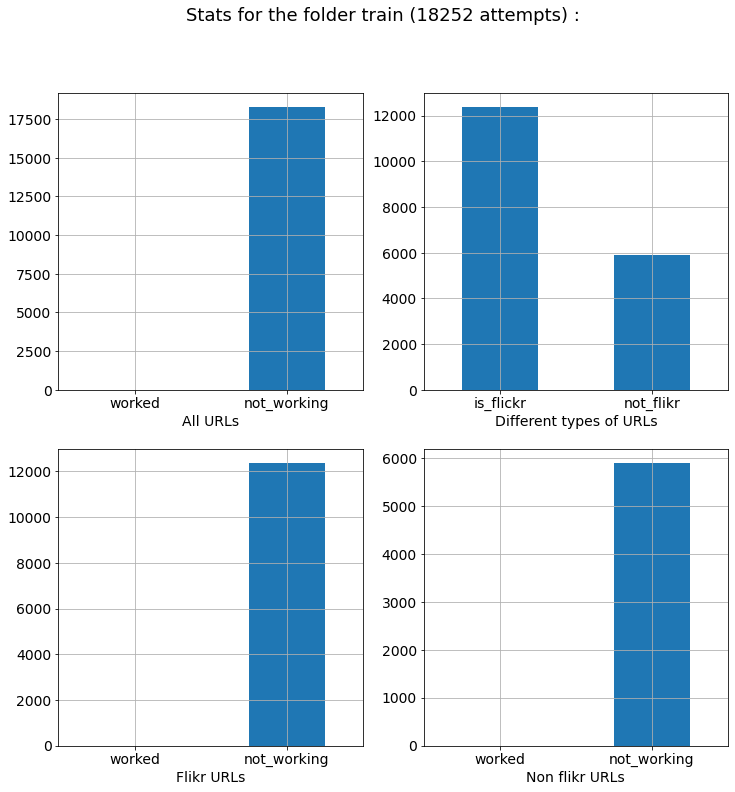# Experimenting with transfer learning for visual categorizationHi! I am Jean-Nicolas Jérémie and the goal of this notebook is to provide a framework to implement (and experiment with) transfer learning on deep convolutional neuronal network (DCNN). In a nutshell, transfer learning allows to re-use the knowlegde learned on a problem, such as categorizing images from a large dataset, and apply it to a different (yet related) problem, performing the categorization on a smaller dataset. It is a powerful method as it allows to implement complex task de novo quite rapidly (in a few hours) without having to retrain the millions of parameters of a DCNN (which takes days of computations). The basic hypothesis is that it suffices to re-train the last classification layers (the head) while keeping the first layers fixed. Here, these networks teach us also some interesting insights into how living systems may perform such categorization tasks.

Based on our previous work, we will start from a VGG16 network loaded from the `torchvision.models` library and pre-trained on the Imagenet dataset wich allows to perform label detection on naturals images for \$K = 1000\$ labels. Our goal here will be to re-train the last fully-Connected layer of the network to perfom the same task but in a sub-set of \$K = 10\$ labels from the Imagenet dataset.

Moreover, we are going to evaluate different strategies of transfer learning:

• VGG General : Substitute the last layer of the pyTorch VGG16 network (\$K = 1000\$ labels) with a new layer build from a specific subset (\$K = 10\$ labels).
• VGG Linear : Add a new layer build from a specific subset (\$K = 10\$ labels) after the last Fully-Connected layer of the the pyTorch VGG16 network.
• VGG Gray : Same architecture as the VGG General network but trained with grayscale images.
• VGG Scale : Same architecture as the VGG General network but trained with images of different size.
• VGG Full : Same architecture as the VGG General network but all the layers are trained (otherwise I trained the last Fully-Connected layer).

In this notebook, I will use the pyTorch library for running the networks and the pandas library to collect and display the results. This notebook was done during a master 2 internship at the Neurosciences Institute of Timone (INT) under the supervision of Laurent Perrinet. It is curated in the following github repo.

## Implementing transfer learning on Vgg16 using pyTorch¶

In our previous work, as the VGG16 network was first trained on the entire dataset of \$K=1000\$ labels, and in order to recover the categorization confidence predicted by the model according to the specific subset of classes (\$K = 10\$ labels) on which it is tested, the output `softmax` mathematical function of the last layer of the network was slightly changed. By assuming that we know a priori that the image belongs to one (and only one) category from the sub-set the probabilities obtained would correspond to a confidence of categorization discriminating only the classes of interest and can be compared to a chance level of \$1 /K\$. This creates another network (which is not retrained) directly based on VGG:

• VGG Subset : Just consider the specific subset (\$K = 10\$ labels) from the last layer of the pyTorch VGG16 network (\$K = 1000\$ labels).

This notebook aims in addition to test this hypothesis. Our use case consists of measuring whether there are differences in the likelihood of these networks during an image recognition task on a sub-set of \$1000\$ classes of the `ImageNet` library, with \$K = 10\$ (experiment 1). Additionally, we will implement some image transformations as up/down-sampling (experiment 2) or transforming to grayscale (experiment 3) to quantify their influence on the accuracy and computation time of each network.

Let's first install requirements

In :
```%pip install --upgrade -r requirements.txt
```
```/usr/lib/python3/dist-packages/secretstorage/dhcrypto.py:15: CryptographyDeprecationWarning: int_from_bytes is deprecated, use int.from_bytes instead
from cryptography.utils import int_from_bytes
/usr/lib/python3/dist-packages/secretstorage/util.py:19: CryptographyDeprecationWarning: int_from_bytes is deprecated, use int.from_bytes instead
from cryptography.utils import int_from_bytes
Defaulting to user installation because normal site-packages is not writeable
Requirement already satisfied: pip in /home/INT/perrinet.l/.local/lib/python3.8/site-packages (from -r requirements.txt (line 4)) (21.3.1)
Requirement already satisfied: matplotlib in /home/INT/perrinet.l/.local/lib/python3.8/site-packages (from -r requirements.txt (line 5)) (3.5.0)
Requirement already satisfied: numpy in /home/INT/perrinet.l/.local/lib/python3.8/site-packages (from -r requirements.txt (line 6)) (1.21.4)
Requirement already satisfied: imageio in /home/INT/perrinet.l/.local/lib/python3.8/site-packages (from -r requirements.txt (line 7)) (2.13.1)
Requirement already satisfied: torch in /home/INT/perrinet.l/.local/lib/python3.8/site-packages (from -r requirements.txt (line 8)) (1.10.0)
Requirement already satisfied: torchvision in /home/INT/perrinet.l/.local/lib/python3.8/site-packages (from -r requirements.txt (line 9)) (0.11.1)
Requirement already satisfied: pandas in /home/INT/perrinet.l/.local/lib/python3.8/site-packages (from -r requirements.txt (line 10)) (1.3.4)
Requirement already satisfied: requests in /home/INT/perrinet.l/.local/lib/python3.8/site-packages (from -r requirements.txt (line 11)) (2.26.0)
Requirement already satisfied: sklearn in /home/INT/perrinet.l/.local/lib/python3.8/site-packages (from -r requirements.txt (line 12)) (0.0)
Requirement already satisfied: scipy in /home/INT/perrinet.l/.local/lib/python3.8/site-packages (from -r requirements.txt (line 13)) (1.7.3)
Requirement already satisfied: seaborn in /home/INT/perrinet.l/.local/lib/python3.8/site-packages (from -r requirements.txt (line 14)) (0.11.2)
Requirement already satisfied: kiwisolver>=1.0.1 in /usr/local/lib/python3.8/dist-packages (from matplotlib->-r requirements.txt (line 5)) (1.3.1)
Requirement already satisfied: cycler>=0.10 in /usr/local/lib/python3.8/dist-packages (from matplotlib->-r requirements.txt (line 5)) (0.10.0)
Requirement already satisfied: setuptools-scm>=4 in /home/INT/perrinet.l/.local/lib/python3.8/site-packages (from matplotlib->-r requirements.txt (line 5)) (6.3.2)
Requirement already satisfied: packaging>=20.0 in /usr/lib/python3/dist-packages (from matplotlib->-r requirements.txt (line 5)) (20.3)
Requirement already satisfied: python-dateutil>=2.7 in /usr/lib/python3/dist-packages (from matplotlib->-r requirements.txt (line 5)) (2.7.3)
Requirement already satisfied: pillow>=6.2.0 in /home/INT/perrinet.l/.local/lib/python3.8/site-packages (from matplotlib->-r requirements.txt (line 5)) (8.4.0)
Requirement already satisfied: pyparsing>=2.2.1 in /usr/lib/python3/dist-packages (from matplotlib->-r requirements.txt (line 5)) (2.4.6)
Requirement already satisfied: fonttools>=4.22.0 in /home/INT/perrinet.l/.local/lib/python3.8/site-packages (from matplotlib->-r requirements.txt (line 5)) (4.28.2)
Requirement already satisfied: typing-extensions in /home/INT/perrinet.l/.local/lib/python3.8/site-packages (from torch->-r requirements.txt (line 8)) (3.10.0.0)
Requirement already satisfied: pytz>=2017.3 in /usr/lib/python3/dist-packages (from pandas->-r requirements.txt (line 10)) (2019.3)
Requirement already satisfied: idna<4,>=2.5 in /usr/lib/python3/dist-packages (from requests->-r requirements.txt (line 11)) (2.8)
Requirement already satisfied: certifi>=2017.4.17 in /usr/lib/python3/dist-packages (from requests->-r requirements.txt (line 11)) (2019.11.28)
Requirement already satisfied: charset-normalizer~=2.0.0 in /home/INT/perrinet.l/.local/lib/python3.8/site-packages (from requests->-r requirements.txt (line 11)) (2.0.7)
Requirement already satisfied: urllib3<1.27,>=1.21.1 in /usr/lib/python3/dist-packages (from requests->-r requirements.txt (line 11)) (1.25.8)
Requirement already satisfied: scikit-learn in /home/INT/perrinet.l/.local/lib/python3.8/site-packages (from sklearn->-r requirements.txt (line 12)) (0.24.2)
Requirement already satisfied: six in /usr/lib/python3/dist-packages (from cycler>=0.10->matplotlib->-r requirements.txt (line 5)) (1.14.0)
Requirement already satisfied: tomli>=1.0.0 in /home/INT/perrinet.l/.local/lib/python3.8/site-packages (from setuptools-scm>=4->matplotlib->-r requirements.txt (line 5)) (1.2.2)
Requirement already satisfied: setuptools in /usr/lib/python3/dist-packages (from setuptools-scm>=4->matplotlib->-r requirements.txt (line 5)) (45.2.0)
Requirement already satisfied: threadpoolctl>=2.0.0 in /home/INT/perrinet.l/.local/lib/python3.8/site-packages (from scikit-learn->sklearn->-r requirements.txt (line 12)) (2.1.0)
Requirement already satisfied: joblib>=0.11 in /home/INT/perrinet.l/.local/lib/python3.8/site-packages (from scikit-learn->sklearn->-r requirements.txt (line 12)) (1.0.1)
Note: you may need to restart the kernel to use updated packages.
```
In :
```%matplotlib inline
# uncommment to re-run training
#%rm -fr models
%mkdir -p DCNN_transfer_learning
%mkdir -p results
%mkdir -p models
```

### Initialization of the libraries/variables¶

Our coding strategy is to build up a small library as a package of scripts in the `DCNN_transfer_learning` folder and to run all calls to that library from this notebook. This follows our previous work in which we benchmarked various DCNNs and which allowed us to select VGG16 network as a good compromise between performance and complexity.

First of all, a `init.py` script defines all our usefull variables like the new labels to learn, the number of training images or the root folder to use. Also, we import libraries to train the different networks and display the results.

In :
```scriptname = 'DCNN_transfer_learning/init.py'
```
In :
```%%writefile {scriptname}

# Importing libraries
import torch
import argparse
import json
import matplotlib
import matplotlib.pyplot as plt
plt.rcParams['xtick.labelsize'] = 18
plt.rcParams['ytick.labelsize'] = 18
import numpy as np
import os
import requests
import time
from math import log

from time import strftime, gmtime
datetag = strftime("%Y-%m-%d", gmtime())

HOST, device = os.uname(), torch.device("cuda" if torch.cuda.is_available() else "cpu")

# to store results
import pandas as pd

def arg_parse():
DEBUG = 25
DEBUG = 1
parser = argparse.ArgumentParser(description='DCNN_transfer_learning/init.py set root')
parser.add_argument("--root", dest = 'root', help = "Directory containing images to perform the training",
default = 'data', type = str)
parser.add_argument("--folders", dest = 'folders', help =  "Set the training, validation and testing folders relative to the root",
default = ['test', 'val', 'train'], type = list)
parser.add_argument("--N_images", dest = 'N_images', help ="Set the number of images per classe in the train folder",
default = [400//DEBUG, 200//DEBUG, 800//DEBUG], type = list)
parser.add_argument("--HOST", dest = 'HOST', help = "Set the name of your machine",
default=HOST, type = str)
parser.add_argument("--datetag", dest = 'datetag', help = "Set the datetag of the result's file",
default = datetag, type = str)
parser.add_argument("--image_size", dest = 'image_size', help = "Set the default image_size of the input",
default = 256)
parser.add_argument("--image_sizes", dest = 'image_sizes', help = "Set the image_sizes of the input for experiment 2 (downscaling)",
default = [64, 128, 256, 512], type = list)
parser.add_argument("--num_epochs", dest = 'num_epochs', help = "Set the number of epoch to perform during the traitransportationning phase",
default = 25//DEBUG)
parser.add_argument("--batch_size", dest = 'batch_size', help="Set the batch size", default = 16)
parser.add_argument("--lr", dest = 'lr', help="Set the learning rate", default = 0.0001)
parser.add_argument("--momentum", dest = 'momentum', help="Set the momentum", default = 0.9)
parser.add_argument("--beta2", dest = 'beta2', help="Set the second momentum - use zero for SGD", default = 0.)
parser.add_argument("--subset_i_labels", dest = 'subset_i_labels', help="Set the labels of the classes (list of int)",
default = [945, 513, 886, 508, 786, 310, 373, 145, 146, 396], type = list)
default = 'imagenet_label_to_wordnet_synset.json', type = str)
default = 'Imagenet_urls_ILSVRC_2016.json', type = str)
parser.add_argument("--model_path", dest = 'model_path', help = "Set the path to the pre-trained model",
default = 'models/re-trained_', type = str)
parser.add_argument("--model_names", dest = 'model_names', help = "Modes for the new trained networks",
default = ['vgg16_lin', 'vgg16_gen', 'vgg16_scale', 'vgg16_gray', 'vgg16_full'], type = list)
return parser.parse_args()

args = arg_parse()
datetag = args.datetag
json_fname = os.path.join('results', datetag + '_config_args.json')
load_parse = False # False to custom the config

with open(json_fname, 'rt') as f:
args.__dict__.update(override)
else:
print(f'Creating file {json_fname}')
with open(json_fname, 'wt') as f:
json.dump(vars(args), f, indent=4)

# matplotlib parameters
colors = ['b', 'r', 'k', 'g', 'm','y']
fig_width = 20
phi = (np.sqrt(5)+1)/2 # golden ratio for the figures :-)

#to plot & display
def pprint(message): #display function
print('-'*len(message))
print(message)
print('-'*len(message))

#DCCN training
print('On date', args.datetag, ', Running benchmark on host', args.HOST, ' with device', device.type)

# Labels Configuration
N_labels = len(args.subset_i_labels)

paths = {}
N_images_per_class = {}
for folder, N_image in zip(args.folders, args.N_images):
paths[folder] = os.path.join(args.root, folder) # data path
N_images_per_class[folder] = N_image
os.makedirs(paths[folder], exist_ok=True)

# gathering labels
labels = []
class_wnids = []
reverse_id_labels = {}
for a, img_id in enumerate(imagenet):
reverse_id_labels[str('n' + (imagenet[img_id]['id'].replace('-n','')))] = imagenet[img_id]['label'].split(',')
labels.append(imagenet[img_id]['label'].split(','))
if int(img_id) in args.subset_i_labels:
class_wnids.append('n' + (imagenet[img_id]['id'].replace('-n','')))

# a reverse look-up-table giving the index of a given label (within the whole set of imagenet labels)
reverse_labels = {}
for i_label, label in enumerate(labels):
reverse_labels[label] = i_label
# a reverse look-up-table giving the index of a given i_label (within the sub-set of classes)
reverse_subset_i_labels = {}
for i_label, label in enumerate(args.subset_i_labels):
reverse_subset_i_labels[label] = i_label

# a reverse look-up-table giving the label of a given index in the last layer of the new model (within the sub-set of classes)
subset_labels = []
pprint('List of Pre-selected classes : ')
# choosing the selected classes for recognition
for i_label, id_ in zip(args.subset_i_labels, class_wnids) :
subset_labels.append(labels[i_label])
print('-> label', i_label, '=', labels[i_label], '\nid wordnet : ', id_)
subset_labels.sort()
```
```Overwriting DCNN_transfer_learning/init.py
```
In :
```%run -int {scriptname}
```
```Creating file results/2021-12-08_config_args.json
On date 2021-12-08 , Running benchmark on host neo-ope-de04  with device cuda
-------------------------------
List of Pre-selected classes :
-------------------------------
-> label 945 = bell pepper
id wordnet :  n02056570
-> label 513 = cornet
id wordnet :  n02058221
-> label 886 = vending machine
id wordnet :  n02219486
-> label 508 = computer keyboard
id wordnet :  n02487347
-> label 786 = sewing machine
id wordnet :  n02643566
-> label 310 = ant
id wordnet :  n03085013
-> label 373 = macaque
id wordnet :  n03110669
-> label 145 = king penguin
id wordnet :  n04179913
-> label 146 = albatross
id wordnet :  n04525305
-> label 396 = lionfish
id wordnet :  n07720875

IPython CPU timings (estimated):
User   :       1.67 s.
System :       3.47 s.
Wall time:       1.26 s.
```

### Download the `train` & `val` dataset¶

In the `dataset.py`, we use an archive of the Imagenet urls (from fall 2011) to populate datasets based on the pre-selected classes listed in the `DCNN_transfer_learning/init.py` file. The following script is inspired by previous work in our group.

In :
```scriptname = 'DCNN_transfer_learning/dataset.py'
```
In :
```%%writefile {scriptname}

from DCNN_transfer_learning.init import *
verbose = False

def clean_list(list_dir, patterns=['.DS_Store']):
for pattern in patterns:
if pattern in list_dir: list_dir.remove('.DS_Store')
return list_dir

import imageio
def get_image(img_url, timeout=3., min_content=3, verbose=verbose):
try:
if (len(img_resp.shape) < min_content):
print(f"Url {img_url} does not have enough content")
return False
else:
if verbose : print(f"Success with url {img_url}")
return img_resp
except Exception as e:
if verbose : print(f"Failed with {e} for url {img_url}")
return False # did not work

import hashlib # jah.
# root folder
os.makedirs(args.root, exist_ok=True)
# train, val and test folders
for folder in args.folders :
os.makedirs(paths[folder], exist_ok=True)

list_urls = {}
list_img_name_used = {}
for class_wnid in class_wnids:
list_urls[class_wnid] =  Imagenet_urls_ILSVRC_2016[str(class_wnid)]
np.random.shuffle(list_urls[class_wnid])
list_img_name_used[class_wnid] = []

# a folder per class in each train, val and test folder
for folder in args.folders :
class_name = reverse_id_labels[class_wnid]
class_folder = os.path.join(paths[folder], class_name)
os.makedirs(class_folder, exist_ok=True)
list_img_name_used[class_wnid] += clean_list(os.listdir(class_folder)) # join two lists

# train, val and test folders
for folder in args.folders :
print(f'Folder \"{folder}\"')

filename = f'results/{datetag}_dataset_{folder}_{args.HOST}.json'
columns = ['img_url', 'img_name', 'is_flickr', 'dt', 'worked', 'class_wnid', 'class_name']
if os.path.isfile(filename):
else:
df_dataset = pd.DataFrame([], columns=columns)

for class_wnid in class_wnids:
class_name = reverse_id_labels[class_wnid]
print(f'Scraping images for class \"{class_name}\"')
class_folder = os.path.join(paths[folder], class_name)
while (len(clean_list(os.listdir(class_folder))) < N_images_per_class[folder]) and (len(list_urls[class_wnid]) > 0):

# pick and remove element from shuffled list
img_url = list_urls[class_wnid].pop()

if len(df_dataset[df_dataset['img_url']==img_url])==0 : # we have not yet tested this URL yet
# Transform URL into filename
# https://laurentperrinet.github.io/sciblog/posts/2018-06-13-generating-an-unique-seed-for-a-given-filename.html
img_name = hashlib.sha224(img_url.encode('utf-8')).hexdigest() + '.png'
tic = time.time()
if img_url.split('.')[-1] in ['.tiff', '.bmp', 'jpe', 'gif']:
if verbose: print('Bad extension for the img_url', img_url)
worked, dt = False, 0.
# make sure it was not used in other folders
elif not (img_name in list_img_name_used[class_wnid]):
img_content = get_image(img_url, verbose=verbose)
worked = img_content is not False
if worked:
if verbose : print('Good URl, now saving', img_url, ' in', class_folder, ' as', img_name)
imageio.imsave(os.path.join(class_folder, img_name), img_content, format='png')
list_img_name_used[class_wnid].append(img_name)
df_dataset.loc[len(df_dataset.index)] = {'img_url':img_url, 'img_name':img_name, 'is_flickr':1 if 'flickr' in img_url else 0, 'dt':time.time() - tic,
'worked':worked, 'class_wnid':class_wnid, 'class_name':class_name}
df_dataset.to_json(filename)
print(f'\r{len(clean_list(os.listdir(class_folder)))} / {N_images_per_class[folder]}', end='\n' if verbose else '', flush=not verbose)

if (len(clean_list(os.listdir(class_folder))) < N_images_per_class[folder]) and (len(list_urls[class_wnid]) == 0):
print('Not enough working url to complete the dataset')
df_dataset.to_json(filename)
```
```Overwriting DCNN_transfer_learning/dataset.py
```
In :
```%run -int {scriptname}
```
```Creating file results/2021-12-01_config_args.json
On date 2021-12-01 , Running benchmark on host neo-ope-de04  with device cuda
-------------------------------
List of Pre-selected classes :
-------------------------------
-> label 945 = bell pepper
id wordnet :  n02056570
-> label 513 = cornet
id wordnet :  n02058221
-> label 886 = vending machine
id wordnet :  n02219486
-> label 508 = computer keyboard
id wordnet :  n02487347
-> label 786 = sewing machine
id wordnet :  n02643566
-> label 310 = ant
id wordnet :  n03085013
-> label 373 = macaque
id wordnet :  n03110669
-> label 145 = king penguin
id wordnet :  n04179913
-> label 146 = albatross
id wordnet :  n04525305
-> label 396 = lionfish
id wordnet :  n07720875
Folder "test"
Scraping images for class "king penguin"
Scraping images for class "albatross"
Scraping images for class "ant"
Scraping images for class "macaque"
Scraping images for class "lionfish"
Scraping images for class "computer keyboard"
Scraping images for class "cornet"
Scraping images for class "sewing machine"
Scraping images for class "vending machine"
Scraping images for class "bell pepper"
Folder "val"
Scraping images for class "king penguin"
Scraping images for class "albatross"
Scraping images for class "ant"
Scraping images for class "macaque"
Scraping images for class "lionfish"
Scraping images for class "computer keyboard"
Scraping images for class "cornet"
Scraping images for class "sewing machine"
Scraping images for class "vending machine"
Scraping images for class "bell pepper"
Folder "train"
Scraping images for class "king penguin"
Scraping images for class "albatross"
Scraping images for class "ant"
Scraping images for class "macaque"
Scraping images for class "lionfish"
Scraping images for class "computer keyboard"
Scraping images for class "cornet"
Scraping images for class "sewing machine"
Scraping images for class "vending machine"
Scraping images for class "bell pepper"

IPython CPU timings (estimated):
User   :       0.28 s.
System :       0.25 s.
Wall time:       0.52 s.
```

Let's plot some statistics for the scrapped images:

In :
```for folder in args.folders :
filename = f'results/{datetag}_dataset_{folder}_{args.HOST}.json'
if os.path.isfile(filename):

df_type = pd.DataFrame({'urls_type': [len(df_dataset[df_dataset['is_flickr']==1]),
len(df_dataset[df_dataset['is_flickr']==0])]},
index=['is_flickr', 'not_flikr'])
df_flikr = pd.DataFrame({'not_flikr': [df_dataset[df_dataset['is_flickr']==0]['worked'].sum(),
(len(df_dataset[df_dataset['is_flickr']==0]) - df_dataset[df_dataset['is_flickr']==0]['worked'].sum())],
'is_flickr': [df_dataset[df_dataset['is_flickr']==1]['worked'].sum(),
(len(df_dataset[df_dataset['is_flickr']==1]) - df_dataset[df_dataset['is_flickr']==1]['worked'].sum())],
'url': [len(df_dataset[df_dataset['worked']==1]), len(df_dataset[df_dataset['worked']==0])]},
index=['worked', 'not_working'])

fig, axes = plt.subplots(figsize=(12,12),nrows=2, ncols=2)
fig.suptitle('Stats for the folder '+ folder + ' (' + str(len(df_dataset)) + ' attempts) :', size = 18)
df_flikr["url"].plot(rot=0, ax=axes[0,0], kind='bar', grid=True, fontsize=14)
axes[0,0].set_xlabel('All URLs', size=14)
df_flikr["not_flikr"].plot(rot=0, ax=axes[1,1], kind='bar', grid=True, fontsize=14)
axes[1,1].set_xlabel('Non flikr URLs', size=14)
df_flikr["is_flickr"].plot(rot=0, ax=axes[1,0], kind='bar', grid=True, fontsize=14)
axes[1,0].set_xlabel('Flikr URLs', size=14)
df_type["urls_type"].plot(rot=0, ax=axes[0,1], kind='bar', grid=True, fontsize=14)
axes[0,1].set_xlabel('Different types of URLs', size=14)

else:
print(f'The file {filename} is not available...')
```Let's show some random images from each label :

In :
```import imageio
folder = 'test'
N_image_i = 5
plot_classes = {}
for class_wnid in class_wnids:
class_name = reverse_id_labels[class_wnid]
class_folder = os.path.join(paths[folder], class_name)
plot_classes[class_name] = os.listdir(class_folder)
x = 0
fig, axs = plt.subplots(len(plot_classes), N_image_i, figsize=(fig_width, fig_width))
for ax, class_name in zip(axs, plot_classes):
for i_image in np.arange(N_image_i):
ax = axs[x][i_image]
path = os.path.join(paths[folder], class_name, plot_classes[class_name][i_image])